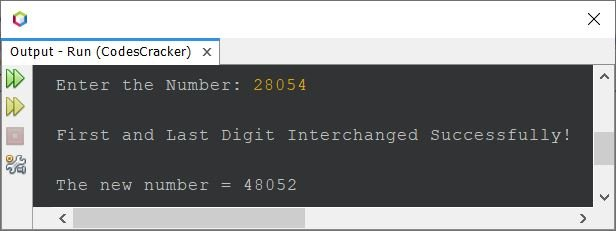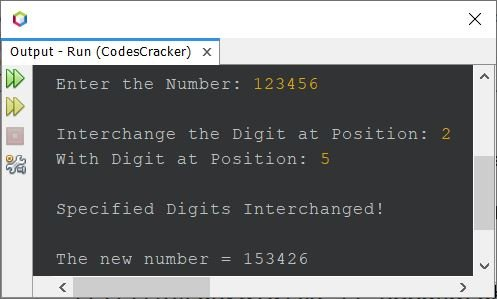# Java Program to Interchange the Digits of a Number

• Interchange the first and last digit of a number using while loop
• Interchange the first and last digit of a number using for loop
• Interchange any two specified digits (by position) of a number

## Swap First and Last Digit of a Number in Java

The question is, write a Java program to swap or interchange the first and last digit of a number. The number must be received by user at run-time. The program given below is its answer. This program is created using while loop:

```import java.util.Scanner;

public class CodesCracker
{
public static void main(String[] args)
{
int num, temp, digits=0, rem, remTemp, rev=0, r, revTemp, digitsTemp;
Scanner scan = new Scanner(System.in);

System.out.print("Enter the Number: ");
num = scan.nextInt();

temp = num;
while(temp>0)
{
digits++;
temp = temp/10;
}
if(digits<2)
{
System.out.println("\nInterchange not possible.");
}
else if(digits==2)
{
temp = num;
while(temp>0)
{
rem = temp%10;
rev = (rev*10) + rem;
temp = temp/10;
}
System.out.println("\nFirst and Last Digit Interchanged Successfully!");
System.out.println("\nThe New Number = " +rev);
}
else
{
temp = num;
while(temp>0)
{
rem = temp%10;
rev = (rev*10) + rem;
temp = temp/10;
}
r = rev;
rev = 0;
temp = num;
digitsTemp = digits;
while(temp>0)
{
remTemp = r%10;
if(digitsTemp==digits || digitsTemp==1)
{
rem = temp%10;
rev = (rev*10) + rem;
}
else
{
rev = (rev*10) + remTemp;
}
r = r/10;
temp = temp/10;
digitsTemp--;
}
System.out.println("\nFirst and Last Digit Interchanged Successfully!");
System.out.println("\nThe new number = " +rev);
}
}
}```

The snapshot given below shows the sample run of above program with user input 28054 as number to swap or interchange its first and last digit:### Interchange First and Last Digit using for Loop

The previous program can also be created using for loop instead of while. I've done some other modifications too, in the program to shorten the code.

```import java.util.Scanner;

public class CodesCracker
{
public static void main(String[] args)
{
int num, temp, digits=0, rev=0, r, digitsTemp;
Scanner scan = new Scanner(System.in);

System.out.print("Enter the Number: ");
num = scan.nextInt();

for(temp=num; temp>0; temp /= 10)
digits++;

if(digits<2)
System.out.println("\nInterchange not possible.");
else if(digits==2)
{
for(temp=num; temp>0; temp /= 10)
rev = (rev*10) + (temp%10);
System.out.println("\nFirst and Last Digit Interchanged Successfully!");
System.out.println("\nThe New Number = " +rev);
}
else
{
for(temp=num; temp>0; temp /= 10)
rev = (rev*10) + (temp%10);
r = rev;
rev = 0;
digitsTemp = digits;
for(temp=num; temp>0; temp /= 10)
{
if(digitsTemp==digits || digitsTemp==1)
rev = (rev*10) + (temp%10);
else
rev = (rev*10) + (r%10);
r = r/10;
digitsTemp--;
}
System.out.println("\nFirst and Last Digit Interchanged Successfully!");
System.out.println("\nThe new number = " +rev);
}
}
}```

And if a number entered by user is always having more than 2 digits. Or if you want a program that works only for more than 2 digit number. Then remove the if-else block of checking the number of digit, to interchange the first and last digit directly. Here is the complete version of the program, works only for 3 or more than 3-digit number:

```import java.util.Scanner;

public class CodesCracker
{
public static void main(String[] args)
{
int num, temp, digits=0, rev=0, r, digitsTemp;
Scanner scan = new Scanner(System.in);

System.out.print("Enter the Number: ");
num = scan.nextInt();

for(temp=num; temp>0; temp /= 10)
digits++;

for(temp=num; temp>0; temp /= 10)
rev = (rev*10) + (temp%10);
r = rev;
rev = 0;
digitsTemp = digits;
for(temp=num; temp>0; temp /= 10)
{
if(digitsTemp==digits || digitsTemp==1)
rev = (rev*10) + (temp%10);
else
rev = (rev*10) + (r%10);
r = r/10;
digitsTemp--;
}
System.out.println("\nFirst and Last Digit Interchanged Successfully!");
System.out.println("\nThe new number = " +rev);
}
}```

## Interchange any Two Digits of a Number in Java

This is the last program, created to interchange any two specified digits of a given number. Since a number may contain two digits with same value. Therefore, this program receives the position of digits, instead of digit's values, to interchange the any two given digits of a number, by position:

```import java.util.Scanner;

public class CodesCracker
{
public static void main(String[] args)
{
int firstPos, secondPos, temp, rev=0, digits=0, i;
Scanner s = new Scanner(System.in);

System.out.print("Enter the Number: ");
int num = s.nextInt();

for(temp=num; temp>0; temp /= 10)
digits++;
int[] arr = new int[digits];

if(digits==1)
System.out.println("\nInterchange not possible!");
else if(digits==2)
System.out.println("\nInterchange with digit-wise is not possible!");
else
{
System.out.print("\nInterchange the Digit at Position: ");
firstPos = s.nextInt();
System.out.print("With Digit at Position: ");
secondPos = s.nextInt();

if(firstPos>digits || secondPos>digits)
System.out.println("\nInvalid Position!");
else
{
for(temp=num; temp>0; temp /= 10)
rev = (rev*10) + (temp%10);
for(i=0; i<digits; i++, rev /= 10)
arr[i] = rev%10;
temp = arr[firstPos-1];
arr[firstPos-1] = arr[secondPos-1];
arr[secondPos-1] = temp;
System.out.println("\nSpecified Digits Interchanged!");
System.out.print("\nThe new number = ");
for(i=0; i<digits; i++)
System.out.print(arr[i]);
}
}
}
}```

The sample run of above program with user input 123456 as number, 2 and 5 as digit's position to interchange the digit at position 2 with the digit at position 5, is shown in the snapshot given below:Java Online Test

« Previous Program Next Program »